## STAM101 :: Lecture 17 :: Latin square design – description – layout – analysis – advantages and disadvantages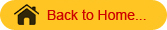Latin Square Design

When the experimental material is divided into rows and columns and the treatments are allocated such that each treatment occurs only once in each row and each column, the design is known as L S D.

In LSD the treatments are usually denoted by A B C D etc.

For a 5 x 5 LSD the arrangements may be

 A B C D E B A E C D C D A E B D E B A C E C D B A Square 1
 B C D E B A D E C C E A B D D C E A B E D B C A Square 2
 A B C D E B C D E A C D E A B D E A B C E A B C D Square  3

Analysis

The ANOVA model for LSD is

Yijk = µ + ri + cj + tk + eijk

ri is the ith row effect
cj is the jth column effect
tk is the kth treatment effect and
eijk is the error term
The analysis of variance table for LSD is as follows:

 Sources of Variation d.f. S S M S F Rows t-1 RSS RMS RMS/EMS Columns t-1 CSS CMS CMS/EMS Treatments t-1 TrSS TrMS TrMS/EMS Error (t-1)(t-2) ESS EMS Total t2-1 TSS

F table value
F [t-1),(t-1)(t-2)] degrees of freedom at 5% or 1%  level of significance

Steps to calculate the above Sum of Squares are as follows:

Correction Factor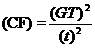Total Sum of Squares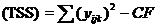Row sum of squares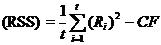Column sum of squares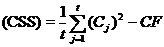Treatment sum of squaresThese results can be summarized in the form of analysis of variance table.

Calculation of SE, SE (d) and CD values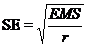where r is the number of rows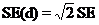.
CD= SE (d). t
where t = table value of t for a specified level of significance and error degrees of freedom
Using CD value the bar chart can be drawn and the conclusion may be written.

• LSD is more efficient than RBD or CRD. This is because of double grouping that will result in small experimental error.

• When missing values are present, missing plot technique can be used and analysed.